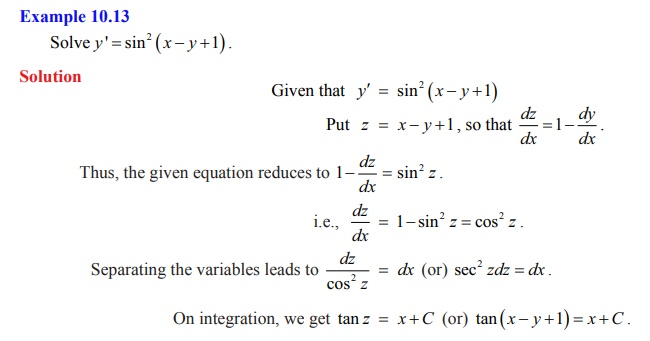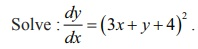Home | | Maths 12th Std | Substitution Method

# Substitution Method

Solution of First Order and First Degree Differential Equations: Substitution Method

## Solution of First Order and First Degree Differential Equations

Substitution Method

Let the differential equation be of the form dy/dx = f (ax + by + c).

(i) If a ≠ 0 and b ≠ 0 , then the substitution ax + by + c = z reduces the given equation to the variables separable form.

(ii) If a = 0 or b = 0 , then the differential equation is already in separable form.### Example 10.16### Solution

To solve the given differential equation, we make the substitution 3x + y + 4 = z.

Differentiating with respect to x, we get dy/dx = dz/dx − 3 . So the given differential equation becomesIn this equation variables are separable. So, separating the variables and integrating, we get the general solution of the given differential equation asTags : Solution of First Order and First Degree Differential Equations | Mathematics , 12th Maths : UNIT 10 : Ordinary Differential Equations
Study Material, Lecturing Notes, Assignment, Reference, Wiki description explanation, brief detail
12th Maths : UNIT 10 : Ordinary Differential Equations : Substitution Method | Solution of First Order and First Degree Differential Equations | Mathematics